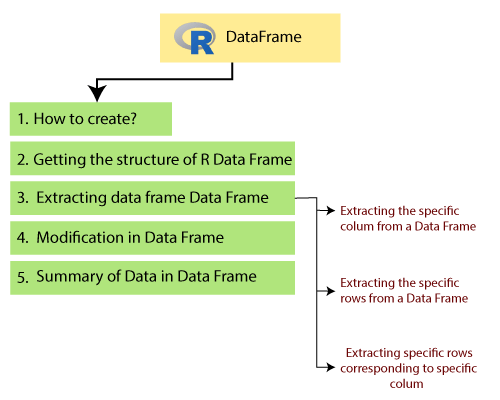# R Data Frame

A data frame is a two-dimensional array-like structure or a table in which a column contains values of one variable, and rows contains one set of values from each column. A data frame is a special case of the list in which each component has equal length.

A data frame is used to store data table and the vectors which are present in the form of a list in a data frame, are of equal length.

In a simple way, it is a list of equal length vectors. A matrix can contain one type of data, but a data frame can contain different data types such as numeric, character, factor, etc.

There are following characteristics of a data frame.

• The columns name should be non-empty.
• The rows name should be unique.
• The data which is stored in a data frame can be a factor, numeric, or character type.
• Each column contains the same number of data items.## How to create Data Frame

In R, the data frames are created with the help of frame() function of data. This function contains the vectors of any type such as numeric, character, or integer. In below example, we create a data frame that contains employee id (integer vector), employee name(character vector), salary(numeric vector), and starting date(Date vector).

Example

``````# Creating the data frame.
emp.data<- data.frame(
employee_id = c (1:5),
employee_name = c("Shubham","Arpita","Nishka","Gunjan","Sumit"),
sal = c(623.3,915.2,611.0,729.0,843.25),

starting_date = as.Date(c("2012-01-01", "2013-09-23", "2014-11-15", "2014-05-11",
"2015-03-27")),
stringsAsFactors = FALSE
)
# Printing the data frame.
print(emp.data)
``````

Output

``````employee_idemployee_namesalstarting_date
1           1       Shubham623.30    2012-01-01
2           2        Arpita915.20    2013-09-23
3           3        Nishka611.00    2014-11-15
4           4        Gunjan729.00    2014-05-11
5          5         Sumit843.25    2015-03-27``````